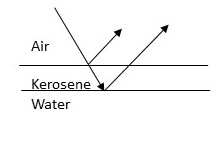# A disabled tanker leaks kerosene (n= 1.20) into the Persian Gulf, creating a large slick on top...

## Question:

A disabled tanker leaks kerosene (n= 1.20) into the Persian Gulf, creating a large slick on top of the water (n = 1.30).

If you are looking straight down from an airplane while the sun is overhead, at a region where its thickness is 460 nm, for which wavelength(s) of visible light in the air (between 400 and 750 nm) is the reflection brightest because of constructive interference? For which wavelength(s) of visible light in air is the reflection dimmest because of destructive interference?## Interference in Thin Films:

Whenever the light hits a thin film of transparent material, the light reflects from the top and bottom surfaces of the material and interferes with itself. The path-length difference between two rays of light determines whether the interference is constructive or destructive. If the two waves are in phase, they interfere constructively and the intensity is maximum. When the path-length difference corresponds to the half-wavelength, the two waves cancel each other and the interference is destructive.

## Answer and Explanation:

The light reflected from kerosene and from water will experience an additional phase shift of {eq}\pi {/eq}, totaling to the {eq}2\pi {/eq} overall phase shift, therefore, we can ignore it in our considerations.

The condition for the constructive interference can be written as follows:

{eq}d n_k = m \lambda {/eq}

Here

• {eq}d = 4.6\cdot 10^{-7} \ m {/eq} is the thickness of the layer of kerosene;
• {eq}n_k = 1.2 {/eq} is the index of refraction of kerosene;
• {eq}m {/eq} is the order of interference;
• {eq}\lambda {/eq} is the wavelength of light;

Solving for the wavelength, we obtain:

{eq}\lambda = \dfrac {d n_k}{m} {/eq}

For the first order maximum (m = 1), we obtain:

{eq}\lambda = \dfrac {4.6\cdot 10^{-7} \ m \cdot 1.2}{1} \approx 5.52\cdot 10^{-7} \ m = \boxed {552 \ nm} {/eq}

The destructive interference occurs when the following condition is met:

{eq}d n_k = \left (m + \dfrac {1}{2} \right ) \lambda {/eq}

For the m = 1 minimum, we get:

{eq}\lambda = \dfrac {2d n_k}{3} {/eq}

Computing, we obtain:

{eq}\lambda = \dfrac {4.6\cdot 10^{-7} \ m \cdot 1.2 \cdot 2}{3} \approx 3.68\cdot 10^{-7} \ m = \boxed {368 \ nm} {/eq}